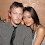## Sunday, January 31, 2016

### 33 | CNN asks, 'Who is Blac Chyna?' on January 31, 2016

Blac = 2+3+1+3 = 9
Chyna = 3+8+7+5+1 = 24
Blac Chyna = 33

Blac = 2+12+1+3 = 18
Chyna = 3+8+25+14+1 = 51
Blac Chyna = 69

Notice this headline comes out on January 31, or 1/31.  Blac Chyna was born on the 131st day of the year, May 11.  Stories by the numbers.

5/11/1988 = 5+11+19+88 = 123
5/11/1988 = 5+11+(1+9+8+8) = 42
5/11/1988 = 5+1+1+1+9+8+8 = 33
5/11/88 = 5+11+88 = 104

Let's hope for her sake, she doesn't end up as a 'Super Bowl 50 ritual' if you know what I mean.

And yet again, it is another tabloid news story that comes back to the Kardashian family.  Surprise, surprise.  They're also a family with 'Super Bowl gematria'.

Kardashian = 2+1+9+4+1+1+8+9+1+5 = 41 (Super Bowl = 41) (Broncos)
Kardashian = 11+1+18+4+1+19+8+9+1+14 = 86 (Symbol) (Broncos)

The Broncos won the Super Bowl 16-years ago today.

1.2.